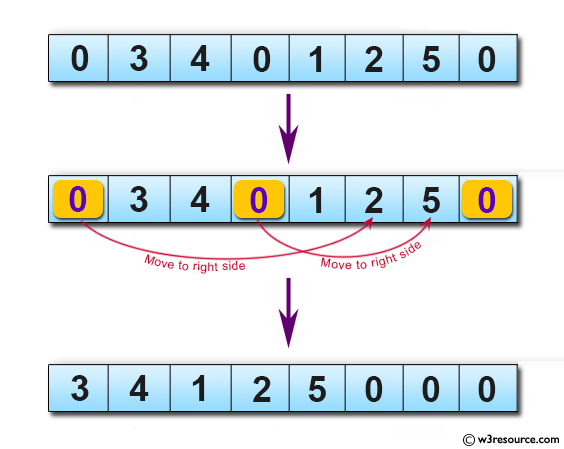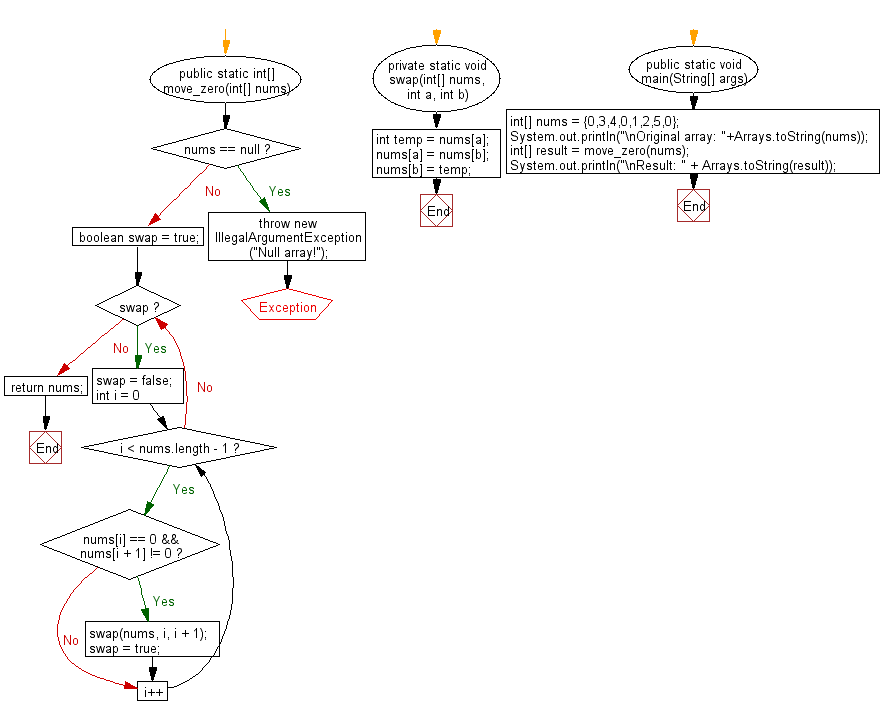﻿ Java programming exercises: Move every zero to the right side of a given array of integers - w3resource# Java Exercises: Move every zero to the right side of a given array of integers

## Java Basic: Exercise-167 with Solution

Write a Java program to move every zero to the right side of a given array of integers.

Pictorial Presentation:Sample Solution:

Java Code:

``````import java.util.*;
public class Solution {
public static int[] move_zero(int[] nums) {
if (nums == null) {
throw new IllegalArgumentException("Null array!");
}
boolean swap = true;
while (swap) {
swap = false;
for (int i = 0; i < nums.length - 1; i++) {
if (nums[i] == 0 && nums[i + 1] != 0) {
swap(nums, i, i + 1);
swap = true;
}
}
}
return nums;
}
private static void swap(int[] nums, int a, int b) {
int temp = nums[a];
nums[a] = nums[b];
nums[b] = temp;
}
public static void main(String[] args) {
int[] nums = {0,3,4,0,1,2,5,0};
System.out.println("\nOriginal array: "+Arrays.toString(nums));
int[] result = move_zero(nums);
System.out.println("\nResult: " + Arrays.toString(result));
}
}
```
```

Sample Output:

```Original array: [0, 3, 4, 0, 1, 2, 5, 0]

Result: [3, 4, 1, 2, 5, 0, 0, 0]
```

Flowchart:Java Code Editor:

What is the difficulty level of this exercise?

﻿

## Java: Tips of the Day

Parsing dates:

```import java.io.*;
import java.util.*;
import java.text.*;

String s = "2001/09/23 14:39";

SimpleDateFormat formatter = new SimpleDateFormat ("yyyy/MM/dd H:mm");
Date d = formatter.parse(s, new ParsePosition(0));
```